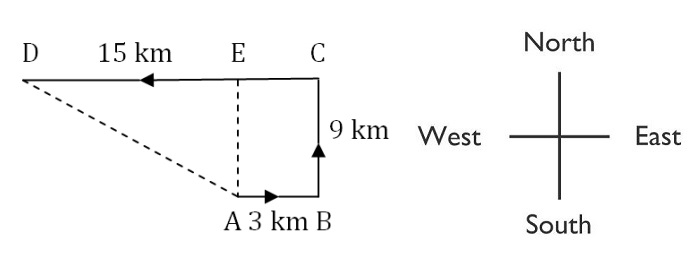## Direction and Distance

#### Direction and Distance

1. A river flows from west to east and on the way turns left and goes in a semi-circle round a hillock, and then turns left at right angle. In which direction is the river finally flowing?

1. There is two ways to draw a figure for the river.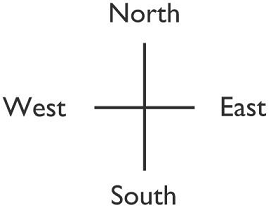##### Correct Option: B

There is two possibilities for drawing a figure for the river.
First possibilities,
First draw a figure for the river in the direction west to east then move to left in circle facing West to East direction and made half circle. Next turn to left at right angle.

Second possibilities,
First draw a figure for the river in the direction west to east then move to left in circle facing East to West direction and made half circle. Next turn to left at right angle.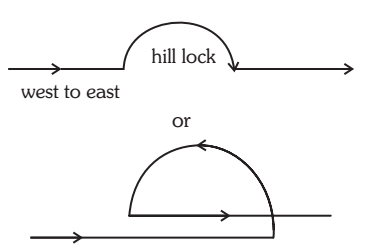1. A man is facing towards west and turns through 45° clockwise, again 180° clockwise and then turns through 270° anti-clockwise. In which direction is he facing now?

1. Draw a figure of man is moving in a direction given in question.##### Correct Option: D

First and second move will be in clockwise and 3rd move will be anticlockwise.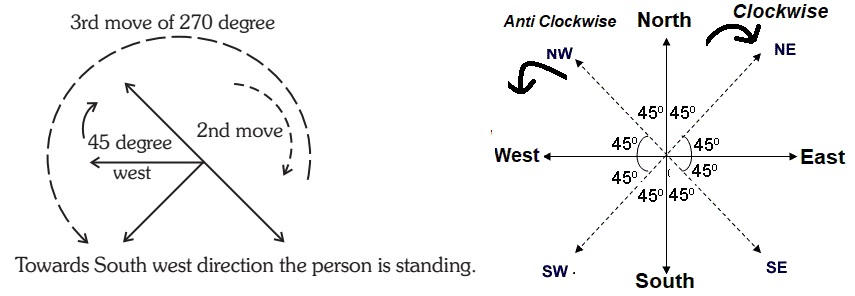1. Namita walks 14 meters towards west, then turns to her right and walks 14 meters and then turns to her left and walks 10 meters. Again turning to her left she walks 14 meters. What is the shortest distance (in meters) between her starting point and the present position?

1. Draw a figure as per given question.##### Correct Option: B

Namita walks 14 meters towards west from starting point S.
(Assume the starting point is S).
Then she turns to her right and walks 14 meters.
And then turns to her left and walks 10 meters.
Again turning to her left she walks 14 meters.

Required answer: DA + SA = 10 + 14 = 24m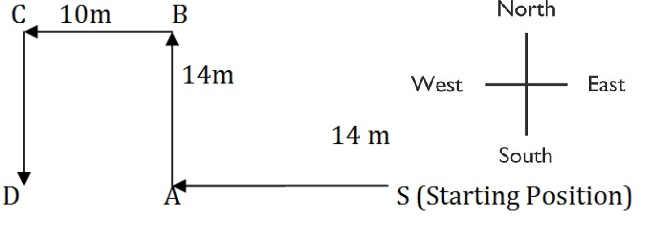1. Amit walked 30 meters towards East, took a right turn and walked 40 meters. Then he took a left turn and walked 30 meters. In which direction is he now from the starting point?

1. Draw a figure with the help of given below direction figure.##### Correct Option: C

The movements of Amit are as shown in Fig (P to Q, Q to R and R to S).
Clearly, his final position is S which is to the South – East from the starting point P.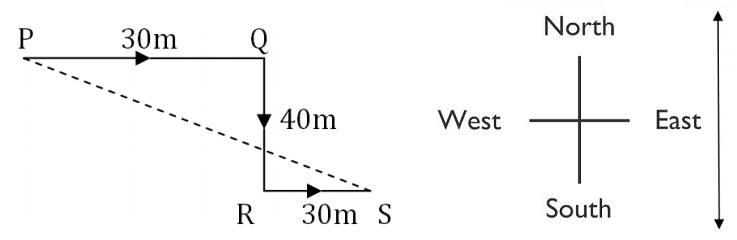1. A person starts from a point A and travels 3 km east - wards to B and then turns left and travels thrice that distance to reach C. He again turns left and travels five times the distance he covered between A and B and reaches his destination D. The shortest distance between the starting point and the destination is?

1. Draw the figure of movement with the help of given below direction graph.
A person starts from a point A and travels 3 km East - Wards to B Then turns left and travels thrice that distance to reach C.
He again turns left and travels five times the distance he covered between A and B and reaches his destination D.##### Correct Option: B

Draw the figure of movement with the help of given below direction graph.
A person starts from a point A and travels 3 km East - Wards to B. Then he turns left and travels 9 km to reach C.
He again turns left and travels 15 km and reaches his destination D.
The movements of the person are as shown in Figure
Clearly, AB = 3 km, BC = 3AB = (3 × 3) km = 9 km, CD = 5AB = (5 × 3) km = 15 km,
Draw AE ? CD.
Then, CE = AB = 3 km
AE = BC = 9 km
DE = (CD – CE) = (15 – 3) km = 12 km.
In triangle AED, AD2 = AE2 + DE2
or AD2 = 92 + 122